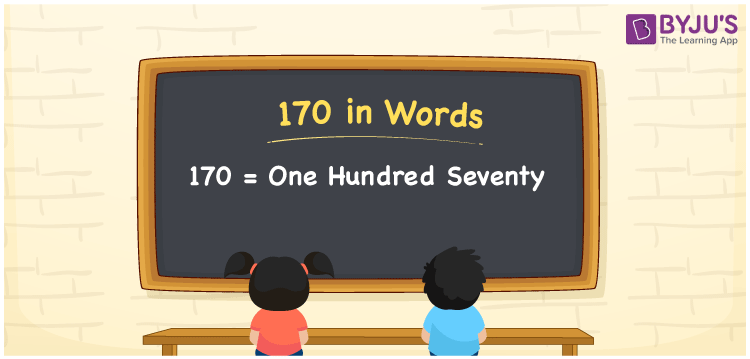# 170 in Words

We can write 170 in words as One Hundred Seventy. It is important to learn the spelling of numbers in the English language to understand and express them in a better way. If you bought a lunch box of Rs. 170, you can say, “I bought a lunch box worth Rupees One Hundred Seventy”. 170 is a cardinal number since it represents a certain amount. In this article, we will learn how to write and read the number 170 in words.

 170 in Words One Hundred Seventy One Hundred Seventy in numerical form 170

## 170 in English Words

English is one of the most common languages that is used in education. Hence, students must learn English to write numbers in words. Therefore, in English, 170 is spelt as One Hundred Seventy.## How to Write 170 in Words?

We can convert the number 170 in word form by knowing the place value of each digit in a number. The table given below is the place value chart for the number 170.

 Hundreds Tens Ones 1 7 0

Hence, we can write the expanded form as:

1 x Hundred + 7 x Ten + 0 x One

= 1 x 100 + 7 x 10 + 0 x 1

= 100 + 70 + 0

= 100 + 70

= 170

= One Hundred Seventy

Thus, 170 in words is written as One Hundred Seventy.

Interesting way of writing 170 in words

1 = One

17 = Seventeen

170 = One Hundred Seventy

Hence, the word form of the number 170 is One Hundred Seventy.

170 is a natural number whose successor is 171 and the predecessor is 169

• 170 in words – One Hundred Seventy
• Is 170 an odd number? – No
• Is 170 an even number? – Yes
• Is 170 a perfect square number? – No
• Is 170 a perfect cube number? – No
• Is 170 a prime number? – No
• Is 170 a composite number? – Yes

## Frequently Asked Questions on 170 in Words

Q1

### How to write 170 in words?

170 in words is written as One Hundred Seventy.
Q2

### Is 170 a perfect square number?

No, 170 is not a perfect square number.
Q3

### What is the place value of 7 in 170?

The place value of 7 in 170 is Tens.